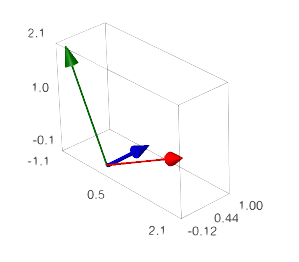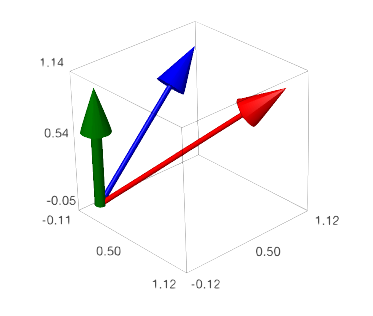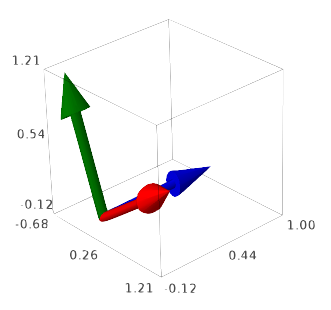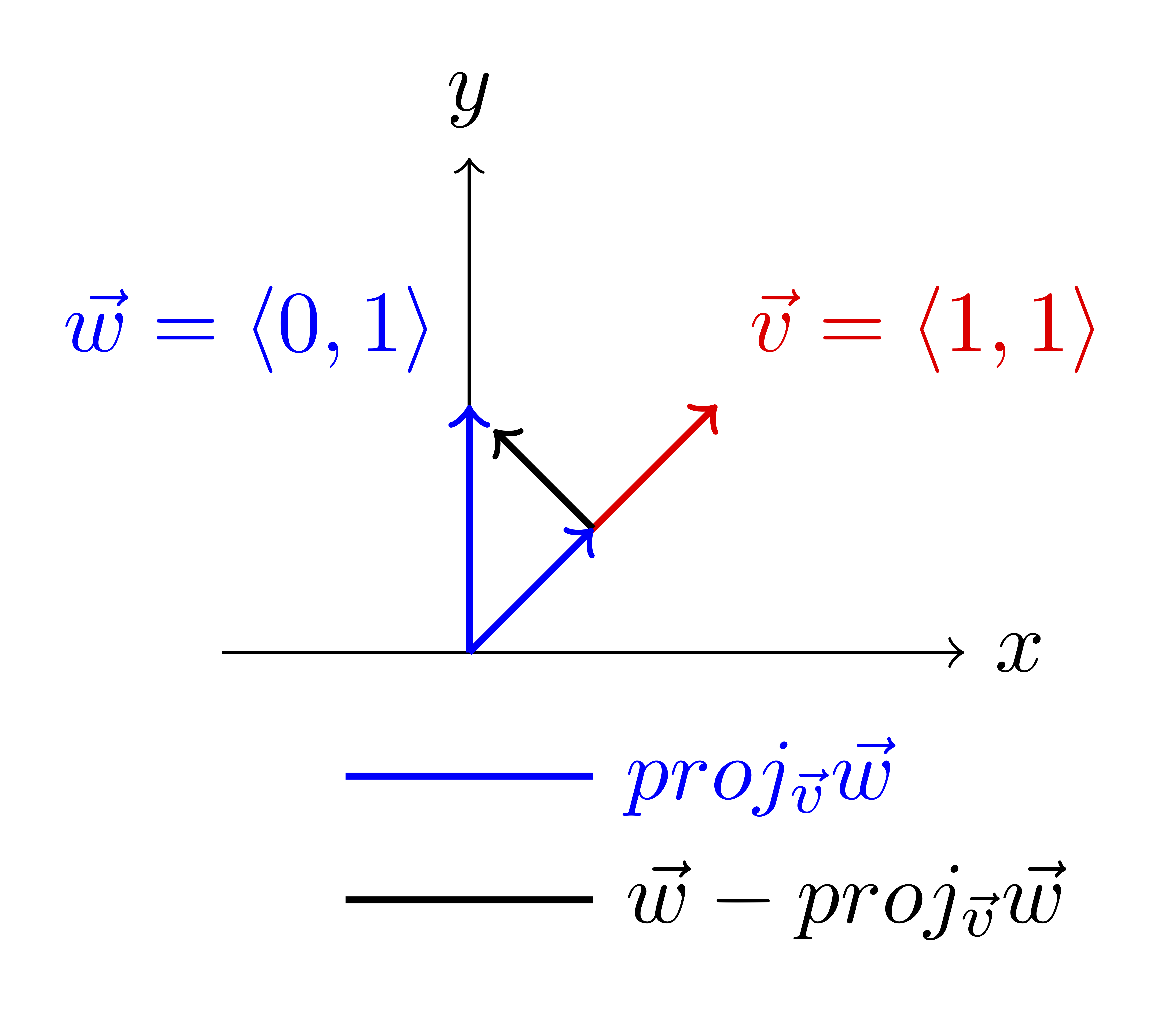## Section3.5Orthogonal Projections and Curve Fitting

### Subsection3.5.1Orthogonal and Orthonormal Bases

###### Definition3.5.1.

A basis $\mathcal{B}$ is said to be an orthogonal basis if each pair of vectors in $\mathcal{B}\text{,}$ $\vec{\beta}_i$ and $\vec{\beta}_j\text{,}$ are orthogonal 1 perpendicular. If in addition $||\vec{\beta}_i||=1$ for all $i$ then we say that the basis is orthonormal.

###### Investigation3.5.1. Orthogonal and Non-Orthogonal Bases.

Consider the bases

\begin{equation*} \mathcal{B}= \left\{ \left[ \begin{array}{r} 2 \\ 0 \\ 1 \end{array} \right], \left[ \begin{array}{r} 0 \\ 1 \\ 0 \end{array} \right], \left[ \begin{array}{r} -1 \\ 0 \\ 2 \end{array} \right] \right\} \end{equation*}and
\begin{equation*} \mathcal{D}= \left\{ \left[ \begin{array}{r} 1 \\ 1 \\ 1 \end{array} \right], \left[ \begin{array}{r} 0 \\ 1 \\ 1 \end{array} \right], \left[ \begin{array}{r} 0 \\ 0 \\ 1 \end{array} \right] \right\}. \end{equation*}Both of the above sets are bases for $\mathbb{R}^3$ but only $\mathcal{B}$ is an orthogonal basis. We can see this because

\begin{align*} \left\lt2,0,1\right\gt\cdot\left\lt0,1,0\right\gt\amp=0\\ \left\lt2,0,1\right\gt\cdot\left\lt-1,0,2\right\gt\amp=0,\ \mbox{ and}\\ \left\lt-1,0,2\right\gt\cdot\left\lt0,1,0\right\gt\amp=0. \end{align*}

However, $\mathcal{D}$ is not since

\begin{equation*} \left\lt1,1,1\right\gt\cdot\left\lt0,1,1\right\gt=2, \end{equation*}

so there is at least one pair of vectors which are not orthogonal.

Further if we replace $\mathcal{B}$ with

\begin{equation*} \mathcal{B'}= \left\{ \left[ \begin{array}{r} \frac{2}{\sqrt{3}} \\ 0 \\ \frac{1}{\sqrt{3}} \end{array} \right], \left[ \begin{array}{r} 0 \\ 1 \\ 0 \end{array} \right], \left[ \begin{array}{r} -\frac{1}{\sqrt{3}} \\ 0 \\ \frac{2}{\sqrt{3}} \end{array} \right] \right\} \end{equation*}we have an orthonormal basis since all the vectors now have length one and are perpendicular. Moreover, since all of these vectors point in the same direction as the vectors of $\mathcal{B}$ we can call this the normalization of $\mathcal{B}\text{.}$

###### Investigation3.5.2. Orthogonalization.

Consider again

\begin{equation*} \mathcal{D}= \left\{ \left[ \begin{array}{r} 1 \\ 1 \\ 1 \end{array} \right], \left[ \begin{array}{r} 0 \\ 1 \\ 1 \end{array} \right], \left[ \begin{array}{r} 0 \\ 0 \\ 1 \end{array} \right] \right\}. \end{equation*}

and suppose that we want to develop an orthonormal basis from this. We will do this a step at a time using the Gram-Schmidt Orthogonalization process.

The process is based on the vector projections we studied before. If $\vec{v}=\left\lt1,1\right\gt$ and $\vec{w}=\left\lt0,1\right\gt\text{,}$ then

\begin{equation*} proj_{\vec{v}}\vec{w}=\frac{\vec{v}\cdot\vec{w}}{\vec{v}\cdot\vec{v}}\vec{v}=\left\lt\frac{1}{2},\frac{1}{2}\right\gt. \end{equation*}

The vector

\begin{equation*} \vec{w}-proj_{\vec{v}}\vec{w}=\left\lt-\frac{1}{2},\frac{1}{2}\right\gt. \end{equation*}

is an orthogonal complement of the projection; it is orthogonal to $\vec{v}$ and we can use it to replace $\vec{w}\text{.}$Consider

\begin{align*} a\vec{v}+b\vec{w}\amp = a\vec{v}+b\vec{w}\\ \amp = a\vec{v}+b\, \left(\vec{w}-proj_{\vec{v}}\vec{w}+proj_{\vec{v}}\vec{w}\right)\\ \amp = a\vec{v}+b\, proj_{\vec{v}}\vec{w}+b\, \left(\vec{w}-proj_{\vec{v}}\vec{w}\right)\\ \amp = a\vec{v}+\frac{b}{2}\vec{v}+b\, \left(\vec{w}-proj_{\vec{v}}\vec{w}\right),\\ \amp = \left(a+\frac{b}{2}\right)\vec{v}+b\, \left(\vec{w}-proj_{\vec{v}}\vec{w}\right), \end{align*}

so we can systematically replace any linear combination of $\vec{v}$ and $\vec{w}$ with a combination of $\vec{v}$ and $\vec{w}-proj_{\vec{v}}\vec{w}\text{.}$ This is the Gram-Schmidt Orthogonalization process.

Some Content!!!

Some Content!!!

Some Content!!!